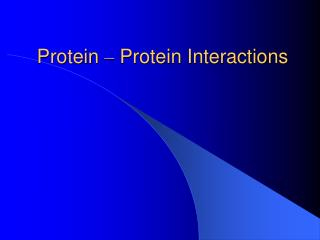DownloadDownload PresentationProtein – Protein Interactions

# Protein – Protein Interactions

Download Presentation## Protein – Protein Interactions

- - - - - - - - - - - - - - - - - - - - - - - - - - - E N D - - - - - - - - - - - - - - - - - - - - - - - - - - -
##### Presentation Transcript

1. Protein – Protein Interactions

2. Implementation/Algorithm • Algorithm approximates the minimum set of domains pairs. • Algorithm needs to be able to choose d-d pairs in an educated, not a randomized fashion. • This educated way can be done using weight functions. Where each domain pair is given a weight, and the largest of the weights is chosen.

3. Plan for Testing • From the available data bank, create training data of different sizes (.01, .25, .5, .75, 1). • Run program which takes domain pairs chosen using training data and our algorithm • Creates all possible P-P interactions and calculates their probability to interact by looking at the protein structure. • Compares calculated P-P interactions with observed interactions. (number of matches, false positive, and false negative p-p interactions) • Calculate fold, specificity, and sensitivity in order to compare to previous research.

4. Prediction Input • Program written which reads in three files: • Protein Structure: • Protein name: p • list of proteins p interacts with • List of domains p contains • Domain Structure: • Name of domain: d • List of proteins which host d • Domain Interaction: • A predicted pair of interacting domains and their interaction probability.

5. Prediction Data Structures • There are four two dimensional vectors: • Protein Interactions: (observed/predicted) • Protein Domains: (observed) • Domain Hosts: (observed) • Domain Interactions: (predicted)

6. Prediction • For all domains Di For all Domains,Dj , Di interacts with For all proteins, Pi, Di is hosted by For all proteins, Pj, Dj is hosted by set Pi interacting with Pj with probability Di Dj interacting • For all proteins Pi For all Proteins Pj For all Domains,Di, Pi contains For all Domains, Dj, Pj contains probabilty Pi_Pj = 1 – PIE(1 – d[Di][Dj] )

7. Metrics for Comparison • By comparing the observed protein interactions with the predicted protein interactions: • False Positive: Number of predicted protein interactions which are not observed experimentally. • False Negative: Number of protein interactions which were observed experimentally but not predicted. • Fold: (number of matching protein pairs between experimental and observed / number of protein pairs with some probability greater than some threshold) / (total number of protein pairs observed / total number of protein pairs) • Specificity: number of matches / total number of predicted interactions • Sensitivity: number of matches / total number of observed interactions

8. Work Finished • Started writing paper • Program prototypes written • Tested using 75% of available data. • Calculated: • False Positives = • False Negatives = • Fold = • Specificity = • Sensitivity =

9. Work in Progress • Writing Paper • Cleaning code • Getting the testing done • Maybe making a few more weight functions • Adding or subtracting weight depending on different assumptions. • Compare with different algorithms/papers out there